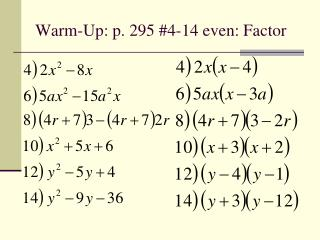DownloadDownload PresentationWarm-Up: p. 295 #4-14 even: Factor

# Warm-Up: p. 295 #4-14 even: Factor

Download Presentation## Warm-Up: p. 295 #4-14 even: Factor

- - - - - - - - - - - - - - - - - - - - - - - - - - - E N D - - - - - - - - - - - - - - - - - - - - - - - - - - -
##### Presentation Transcript

1. Warm-Up: p. 295 #4-14 even: Factor

2. Homework Questions?

3. PSAT Questions?

4. Solving Quadratic Equations Lesson 5.3, Part 2

5. Essential Question • How can we use factoring in order to solve quadratic equations?

6. Solving Quadratic Equations • There must be an = to be an equation • Move everything to one side so that one side = 0 • Use the zero product property • If pq = 0, then p = 0 or q = 0 • Set each factor equal to zero • Solve each equation to find up to two solutions.

7. Example 1 – Solve for x

8. Example 2 – Solve for x

9. You-Trys

10. Warm-Up: October 16, 2012 Solve for x by factoring and zero product property

11. Quiz Thursday • Quiz on Factoring • 10 questions, all free response • 15 minute time limit • Will be included in your progress report grade

12. Homework Questions?

13. PSAT Questions?

14. Finding Zeros of Quadratic Functions • Given f(x) = ax2 + bx + c • The zeros are where f(x) = 0 • Where the parabola crosses the x-axis Zeros

15. They all mean the same… • Roots • Zeros • x-intercepts • They all mean set f(x)=0 and solve for x

16. Example 3 • Find the zeros of g(x) = 6x2 + 20x – 16

17. You-Try #4 • Find the zeros of f(n) = n2 – n – 30

18. Assignment • Page 296-297 #69-87 odd, 101 • Check answers in back of book after you complete the problems. Fix any incorrect problems.

19. October 17, 2012 • Factor the following:

20. Warm-Up: October 18, 2012 • Factor the following:

21. Earthquake Drill Today at 10:18 • Duck and Cover when you hear the bell • After 30-60 seconds another bell will ring. This is the signal to evacuate. • Take all your possessions with you. You will go directly to 4th period after the drill. • You will follow Mr. Szwast to the utility field. • You must stay with the class on the field in single file line.